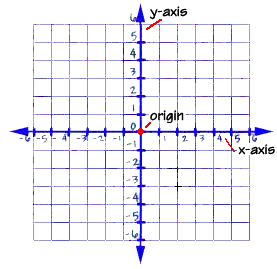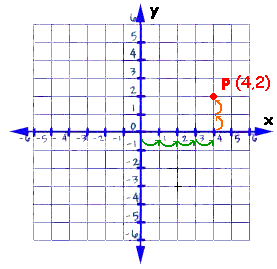Home    |    Teacher    |    Parents    |    Glossary    |    About UsThe idea of graphing with coordinate axes dates all the way back to Apollonius in the second century B.C. Rene Descartes, who lived in the 1600s, gets the credit for coming up with the two-axis system we use today. The story goes that he lay in bed and watched flies crawling over tiles on the ceiling. He realized that he could describe a fly's position using the intersecting lines of the tiles. The system is often called the "Cartesian coordinate system" in his honor.

When working with equations that have two variables, the coordinate plane is an important tool. It's a way to draw pictures of equations that makes them easier to understand.

To create a coordinate plane, start with a sheet of graph or grid paper. Next, draw a horizontal line. This line is called the x-axis and is used to locate values of x. To show that the axis actually goes on forever in both directions, use small arrowheads at each end of the line. Mark off a number line with zero in the center, positive numbers to the right, and negative numbers to the left.Next draw a vertical line that intersects the x axis at zero. This line is called the y-axis and is used to locate the values of y. Mark off a number line with zero in the center, positive numbers going upwards, and negative numbers going downwards. The point where the x and y axes intersect is called the origin. The origin is located at zero on the x axis and zero on the y axis.

Locating Points Using Ordered Pairs
We can locate any point on the coordinate plane using an ordered pair of numbers like the example shown here, the ordered pair 4 and 2 (point P). We call the ordered pair the coordinates of the point. The coordinates of a point are called an ordered pair because the order of the two numbers is important.The first number in the ordered pair is the x coordinate. It describes the number of units to the left or right of the origin. The second number in the ordered pair is the y coordinate. It describes the number of units above or below the origin. To plot a point, start at the origin and count along the x axis until you reach the x coordinate, count right for positive numbers, left for negative. Then count up or down the number of the y coordinate (up for positive, down for negative.)

For example, to graph the point P above, with the ordered pair (4, 2) we count right along the x axis 4 units, and then count up 2 units. Be careful to always start with the x axis, the point (4,2) is very different than the point (2,4)!

To make it easy to talk about where on the coordinate plane a point is, we divide the coordinate plane into four sections called quadrants.Points in Quadrant 1 have positive x and positive y coordinates.
Points in Quadrant 2 have negative x but positive y coordinates.
Points in Quadrant 3 have negative x and negative y coordinates.
Points in Quadrant 4 have positive x but negative y coordinates.

 Homework Help | Algebra | Graphing Equations and InequalitiesEmail this page to a friendSearch·  The coordinate plane·  Slope and y-intercept·  Graphing linear     equationsFirst Glance In Depth Examples WorkoutThe coordinate plane